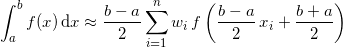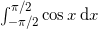by on October 4, 2011

Let us explore the derivation of the Gauss-Legendre quadrature’s abscissae and weights, accurate to any desired number of decimal places, and how this is implemented in the Multiprecision Computing Toolbox for MATLAB.

The User’s Guide section of our website shows an example of how to convert MATLAB programs to enable computation of the Gauss-Legendre quadrature’s abscissae and weights with arbitrary precision. In the latest version of the toolbox 3.1.4.1526, we decided to take this one step further.

We have implemented the calculation of the Gauss-Legendre quadrature’s coefficients in multiple precision as a native module of the toolbox. Now, Gaussian abscissae and weights can be computed with any desired degree of accuracy in MATLAB by a direct call to the mp.GaussLegendre(N) function from the Multiprecision Computing Toolbox.

Here is an example of the new function usage – computation of quadrature’s coefficients accurate to 50 decimal places:

>> mp.Digits(50); >> [x,w] = mp.GaussLegendre(10); >> x' -0.97390652851717172007796401208445205342826994669238 -0.86506336668898451073209668842349304852754301496533 -0.67940956829902440623432736511487357576929471183481 -0.43339539412924719079926594316578416220007183765625 -0.14887433898163121088482600112971998461756485942069 0.14887433898163121088482600112971998461756485942069 0.43339539412924719079926594316578416220007183765625 0.67940956829902440623432736511487357576929471183481 0.86506336668898451073209668842349304852754301496533 0.97390652851717172007796401208445205342826994669238   >> w' 0.06667134430868813759356880989333179285786483432016 0.14945134915058059314577633965769733240255663966943 0.21908636251598204399553493422816319245877187052268 0.26926671930999635509122692156946935285975993846088 0.29552422471475287017389299465133832942104671702685 0.29552422471475287017389299465133832942104671702685 0.26926671930999635509122692156946935285975993846088 0.21908636251598204399553493422816319245877187052268 0.14945134915058059314577633965769733240255663966943 0.06667134430868813759356880989333179285786483432016

The newly computed values can be subsequently used to perform high precision numerical integration. Using the Gaussian quadrature formula,we obtain an accurate 50 digit approximation ofwith the N=20 rule:

>> mp.Digits(50); >> a = mp('-pi/2'); >> b = mp('pi/2'); >> d = (b-a)/2; >> c = (b+a)/2;   >> [x,w] = mp.GaussLegendre(20); >> d*sum(w.*cos(d.*x+c)) 2.00000000000000000000000000000000000000000000000000

In addition, the coefficients derivation algorithm is now heavily optimized, enabling high order quadrature computation:

>> mp.Digits(50);   >> tic; [x,w] = mp.GaussLegendre(10); toc; Elapsed time is 0.009176 seconds.   >> tic; [x,w] = mp.GaussLegendre(100); toc; Elapsed time is 0.053641 seconds.   >> tic; [x,w] = mp.GaussLegendre(1000); toc; Elapsed time is 4.146183 seconds.

The newly introduced function in Multiprecision Computing Toolbox shows a significant boost in performance when compared to the previously mentioned MATLAB program. The program computes 30 times faster than before for N=32 (this actually grows exponentially with increasing quadrature order):

>> mp.Digits(300); % Use 300 digits accuracy for N=32 quadrature >> tic; [x,w] = mp.GaussLegendre(32); toc; % Native multiprecision function from toolbox Elapsed time is 0.033350 seconds.   >> N = 32; >> tic; [x,w] = gauss(mp(N,300)); toc; % MATLAB program converted by toolbox Elapsed time is 0.937171 seconds.

The validity of the calculated coefficients was checked against proven publicly available tables, as well as by the verification of their mathematical properties.

*C++ source code was borrowed and modified from Gauss-Legendre Numerical Integration website.